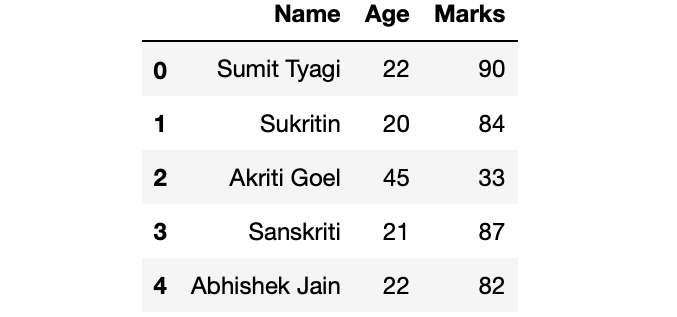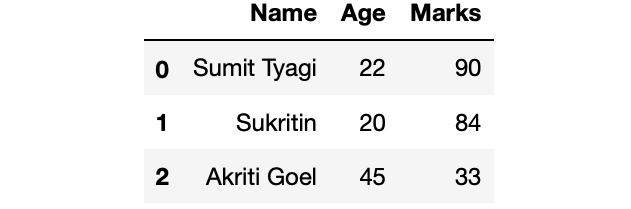Related Articles

# Get first n records of a Pandas DataFrame

• Last Updated : 05 Aug, 2020

Let us see how to fetch the first n records of a Pandas DataFrame. Lets first make a dataframe :

 `# Import Required Library``import` `pandas as pd`` ` `# Create a dictionary for the dataframe``dict` `=` `{``'Name'` `: [``'Sumit Tyagi'``, ``'Sukritin'``,``                  ``'Akriti Goel'``, ``'Sanskriti'``,``                  ``'Abhishek Jain'``],``        ``'Age'``:[``22``, ``20``, ``45``, ``21``, ``22``],``        ``'Marks'``:[``90``, ``84``, ``33``, ``87``, ``82``]}`` ` `# Converting Dictionary to Pandas Dataframe``df ``=` `pd.DataFrame(``dict``)`` ` `# Print Dataframe``print``(df)`

Output :Method 1 : Using `head()` method. Use pandas.DataFrame.head(n) to get the first n rows of the DataFrame. It takes one optional argument n (number of rows you want to get from the start). By default n = 5, it return first 5 rows if value of n is not passed to the method.

 `# Getting first 3 rows from df``df_first_3 ``=` `df.head(``3``)`` ` `# Printing df_first_3``print``(df_first_3)`

Output :Method 2 : Using `pandas.DataFrame.iloc()`. Use pandas.DataFrame.iloc() to get the first n rows. It is similar to the list slicing.

 `# Getting first 3 rows from df``df_first_3 ``=` `df.iloc[:``3``]`` ` `# Printing df_first_3``print``(df_first_3)`

Output :Method 3 : Display first n records of specific columns

 `# Getting first 2 rows of columns Age and Marks from df``df_first_2 ``=` `df[[``'Age'``, ``'Marks'``]].head(``2``)`` ` `# Printing df_first_2``print``(df_first_2)`

Output :Method 4 : Display first n records from last n columns. Display first n records for the last n columns using `pandas.DataFrame.iloc()`

 `# Getting first n rows and last n columns from df``df_first_2_row_last_2_col ``=` `df.iloc[:``2``, ``-``2``:]`` ` `# Printing df_first_2_row_last_2_col``print``(df_first_2_row_last_2_col)`

Output :Attention geek! Strengthen your foundations with the Python Programming Foundation Course and learn the basics.

To begin with, your interview preparations Enhance your Data Structures concepts with the Python DS Course. And to begin with your Machine Learning Journey, join the Machine Learning – Basic Level Course

My Personal Notes arrow_drop_up